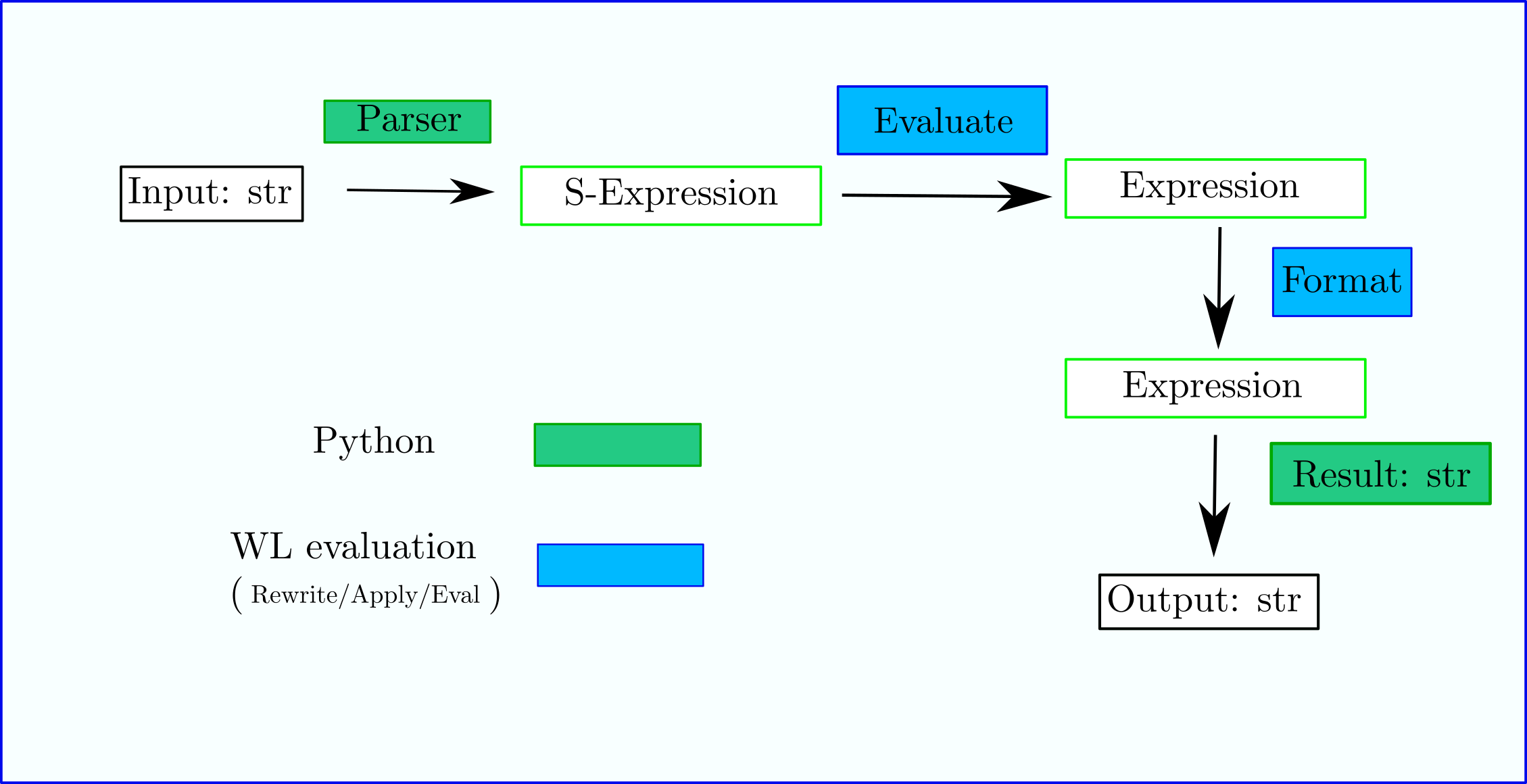# Session¶

A Mathics Session consists of a Definitions object and an Evaluation object.

We describe these two components next.

A diagram of a single step in the Read/Eval/Print Loop (REPL) is shown in the diagram below:## Definitions¶

Front-ends like (django, mathicsscript, mathics, MathicsSession) create a Definitions object which initially has definitions of the thousand or so standard Mathics Builtins. Front ends can customize these definitions. Usually more variables are added under the Settings name space. For example, for mathicsscript there is a variable containing the syntax-highlighting style to use; it can be altered. Also there is a list of all possible syntax styles styles which is not alterable and not in the Settings namespace. For Django, there is a Boolean setting indicating whether or not to use a Sans-Serif font.

## session.evaluate() at a High Level¶

In order to process input requests, an Evaluation object needs to be created using some set of definitions. (Right now a new evaluation object is created for each top-level expression, but this might not be needed, and the prior object might be reused.)

This session evaluation object has a scan-and-parse method which is passed some sort of I/O handle to read from. The result of that is an M-expression described in AST.

This M-expression result is then passed to the evaluate() method of the evaluation object. evaluate() is an apply/eval process that is typically found functional languages; the “apply” phase here covers term-rewriting application and function-symbol application.

In the rewrite/apply/eval process, rules, symbols and function definitions can get altered. A front end will want the changed definitions to persist in a Mathics session while the Evaluation object may or may not.

The direct or return result of rewrite/apply/eval is a Mathics Expression for the input.

For example if the input expression was parsed to the M-expression Plus[1, 2] the output Expression would be 3. Recall that numbers and symbols are expressions too.

This result type, Mathics Expression, differs from the input M-Expression in that symbols found in the Expression may be bound and various properties may be attached to the expression and its subexpressions. For example we may tag that resulting expression with a property that it is numeric such as in the example above where the result Expression was 3.

In those cases where nothing can be filled in, the result may be an M-Expression. And here it can often is same M-Expression that was input.

Here is a simple example showing how to do evaluation from a session in Python using session.evaluate():

\$ python
Python 3.8.10 ..
>>> from mathics.session import MathicsSession
>>> session = MathicsSession()
>>> graph_circle = session.evaluate("Graph3D[Circle[]]")
>>> graph_circle
<Expression: GlobalGraph3D[SystemCircle[SystemList[0, 0]]]>


In the above example, the input Graph3D[Circle[]] is changed, but not that much:

• Namespaces are filled in from the abbreviated variables names. So we have GlobalGraph3D instead of Graph3D and SystemCircle instead of Circle

• Rewrite rules have been applied. Here, it it is just to take the empty parameter list for Circle, [], and expand that into a list, SystemList[0, 0]. Note that internally no nice abbreviations like {0, 0} are used for the replaced output list. Expansion here has the effect of filling in the default value for a circle: a point whose center is at 0, 0.

We will come back to this example in the next section on formatting.

The pipeline sequence of operations: tokenize input, parse tokens, and evaluate is common, and is done continuously inside a REPL. So there is a method on the evaluation method called parse_evaluate() that does all 3 of these things.

The result from a top-level parse_evaluate() is a special Result kind of object containing:

out:

a Python list containing all the messages and printed strings produced

line_no:

the last line number for how far in the input progressed. This is most useful if there was an error.

result:

a Python object containing the formatted version of the result of the evaluation

last_eval:

the last result of the evaluation (an M-Expression), without formatting.

After reading in an expression, parsing it and evaluating it, a front end will typically will want to show the results.

## Expression.format()¶

Here we describe the formatting process that produces result from the Expression in last_eval.

Expressions need to be wrapped in some sort of “Form”, like TeXForm or MathMLForm. This is done using the format() method of the expression object. This goes through the rewrite/apply/eval process producing a Mathics Expression where “Box”ing rules have been applied at various points in the expression; boxing functions associated with expression objects, direct the boxing process.

Continuing using the example in the last section:

>>> graph_circle
<Expression: GlobalGraph3D[SystemCircle[SystemList[0, 0]]]>
>>>  graph_circle.format(session.evaluation, "TeXForm")
<Expression: SystemRowBox[SystemList["\text{Graph3D}\left[\text{Circle}\left[\left\{0,0\right\}\right]\right]"]]>
>>> graph_circle.format(session.evaluation, "MathMLForm")
<Expression: SystemRowBox[SystemList["<math display="block"><mrow><mi>Graph3D</mi> <mo>[</mo> <mrow><mi>Circle</mi> <mo>[</mo> <mrow><mo>{</mo> <mrow><mn>0</mn> <mo>,</mo> <mn>0</mn></mrow> <mo>}</mo></mrow> <mo>]</mo></mrow> <mo>]</mo></mrow>[/itex]"]]>
>>>


Notice in the above that format() was passed session.evaluation. This gives the formatting the ability not just to query the environment outside of what was passed inside the graph_circle expression, but it also allows the fomatting to call back Mathics to perform additional calculations. For example, it is conceivable that a particular formatter might want to know on what plain a particular polygon lies on, and Mathics might be able to get the answer to that.

This box expression is at the end converted into a string by means of the method boxes_to_text()` on the form-boxed-formatted Expression, and is what at the end will be processed and shown in the front end.